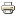# The equivalence between two-person symmetric games and decision problemsWorking paper
Author/s:
Ismail M.S.
Issue number:
23.2014
Series:
Maastricht University, Graduate School of Business and Economics (GSBE) Research Memorandum
Year:
2014
We illustrate an equivalence between the class of two-person symmetric games and the class of decision problems with a complete preference relation. Moreover, we show that a strategy is an optimal threat strategy Nash, 1953 in a two-person symmetric game if and only if it is a maximal element in its equivalent decision problem. In particular, a Nash equilibrium in a two-person symmetric zero-sum game and a pair of maximal elements in its equivalent decision problem coincide. In addition, we show that a two-person symmetric zero-sum game can be extended to its von Neumann-Morgenstern vN-M mixed extension if and only if the extended decision problem satisfies the SSB utility Fishburn, 1982 axioms. Furthermore, we demonstrate that a decision problem satisfies vN-M utility if and only if its equivalent symmetric game is a potential game. Accordingly, we provide a formula for the number of linearly independent equations in order for the independence axiom to be satisfied which grows quadratically as the number of alternatives increase.
Developed by Paolo Gittoi